Welcome to the Parallax Discussion Forums, sign-up to participate.

# Appcon RF1276 Point to Point P2P Failure over time

Configuration of RF1276: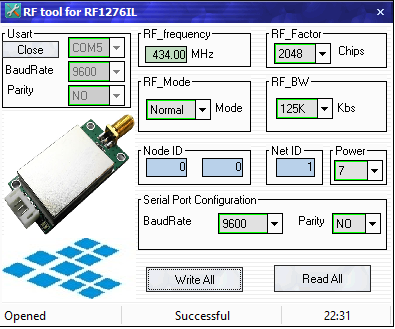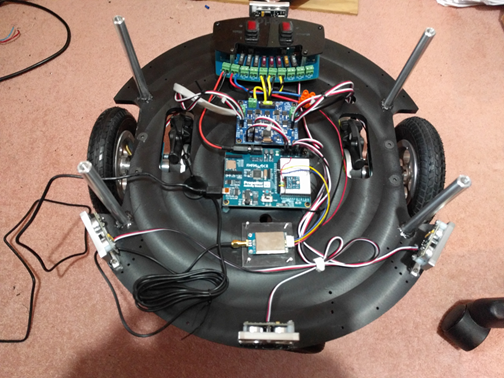Controller using Propeller: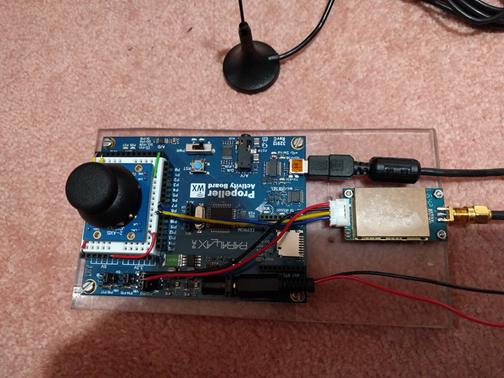Output: Arlo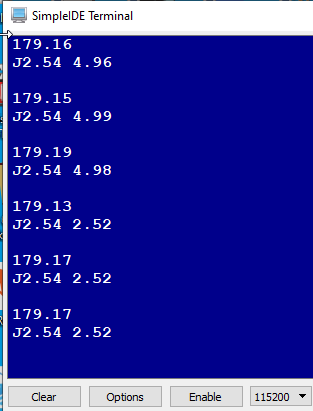Output: controllerCode for Arlo:
```/*
mobile.c

Demonstrates using full duplex serial communication to exchange information.
https://www.appconwireless.com/showpro.php?id=94
http://learn.parallax.com/tutorials/language/propeller-c/propeller-c-simple-protocols/full-duplex-serial
https://github.com/parallaxinc/Simple-Libraries/blob/master/Learn/Examples/Protocols/XBee UART Command Mode Example.c
*/

#include "simpletools.h"
#include "fdserial.h"
#include "compass3d.h"

void compass(void *par);
fdserial *robot;
fdserial *term;
unsigned int stack[44 + 128];
static volatile char dataIn;

int main()
{
robot = fdserial_open(9, 8, 0, 9600);  // fdserial *fdserial_open(int rxpin, int txpin, int mode, int baudrate)
pause(1000);
term = fdserial_open(31, 30, 0, 115200);
pause(1000);
fdserial_rxFlush(robot);
cogstart(compass, NULL, stack, sizeof(stack));
int n = 0;
char c;

while(1)
{
for (int i = 0; i < 11; i++)
{
dataIn[i] = '\0';
}
n = 0;
c = fdserial_rxChar(robot);
if(c != -1)
{
while (n < 10)
{
dataIn[n] = c;
n++;
c = fdserial_rxChar(robot);
if (c == -1 || c == '\n')
break;
}
dataIn = '\0';
dprint(term, "J%s\n", dataIn);
pause(1000);
}
else
{
//    dprint(term, "0XAF 0XAF 0X00 0X00 0XAF 0X80 0X20 0X02 00 00 CS 0X0D 0X0A");
fdserial_rxFlush(robot);
}
}
}

void compass(void *par)
{
int x, y, z;                                // Declare x, y, & z axis variables

i2c *bus = i2c_newbus(3, 2, 0);             // New I2C bus SCL=P3, SDA=P2
compass_init(bus);                          // Initialize compass on bus.

while(1)                                    // Repeat indefinitely
{
compass_read(bus, &x, &y, &z);            // Compass vals -> variables
float fy = (float) y;                     // Ints to floats
float fx = (float) x;
float heading = atan2(fy, fx) * 180.0/PI; // Calculate heading with floats
if(heading < 0.0)                         // Convert -180...+180 to 0...360
//    fdserial_txFlush(robot);
}
}
```
Code for Controller:
```/*
controller.c

Demonstrates using full duplex serial communication to exchange information.
https://www.appconwireless.com/showpro.php?id=94
http://learn.parallax.com/tutorials/language/propeller-c/propeller-c-simple-protocols/full-duplex-serial
https://github.com/parallaxinc/Simple-Libraries/blob/master/Learn/Examples/Protocols/XBee UART Command Mode Example.c
*/

#include "simpletools.h"
#include "fdserial.h"

void joystick(void *par);
fdserial *robot;
fdserial *term;
unsigned int stack[44 + 128];
static volatile char dataIn;

int main()
{
robot = fdserial_open(9, 8, 0, 9600);  // fdserial *fdserial_open(int rxpin, int txpin, int mode, int baudrate)
pause(1000);
term = fdserial_open(31, 30, 0, 115200);
pause(1000);
cogstart(joystick, robot, stack, sizeof(stack));
int n = 0;
char c;

while(1)
{
for (int i = 0; i < 11; i++)
{
dataIn[i] = '\0';
}
n = 0;
c = fdserial_rxChar(robot);
if(c != -1)
{
while (n < 10)
{
dataIn[n] = c;
n++;
c = fdserial_rxChar(robot);
if (c == -1 || c == '\n')
break;
}
dataIn = '\0';
dprint(term, "C%s\n", dataIn);
pause(1000);
}
}
}

void joystick(void *par)
{
//  pause(1000);                                      // Wait 1 s for Terminal app
adc_init(21, 20, 19, 18);                         // CS=21, SCL=20, DO=19, DI=18

float lrV, udV;                                   // Voltage variables

while(1)                                          // Loop repeats indefinitely
{
udV = adc_volts(2);                             // Check A/D 2
lrV = adc_volts(3);                             // Check A/D 3

//pause(1000);                                     // Wait 1/10 s
dprint(robot, "%.2f %.2f\n", udV, lrV);       // Display voltage
pause(1000);                                     // Wait 1/10 s
dprint(term, "%.2f %.2f\n", udV, lrV);       // Display voltage
pause(1000);                                     // Wait 1/10 s
//    dprint(robot, "%.2f\n", lrV);       // Display voltage
//    pause(1000);                                     // Wait 1/10 s
}
}
```
At first I had only one or two lines of output but by using dprint(robot, "%.2f %.2f\n", udV, lrV); and dprint(term, "%.2f %.2f\n", udV, lrV); as above the output continues for a short period but finally transmission stops at both ends.
Perhaps there is something more I need to do with the fdserial library?
Many thanks,
Kevin.

• I have modified the RF1276 configuration: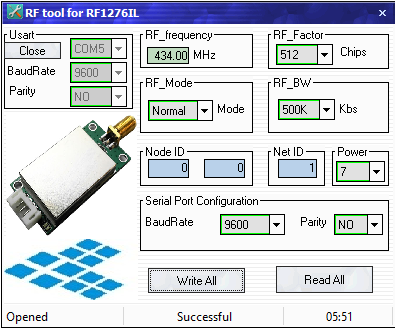The default setting RF_BW 125Khz and RF_factor 2048 means the air data rate is 600bps. We recommend you set the RF_Factor 512, RF_BW 500Khz to try.
RF_Factor: Lora spreading factor. Larger value means higher sensitivity but longer air transmission time
RF_Factor 1 byte 7=128, 8=256, 9=512, 10=1024, 11=2048, 12=4096
RF_BW: Lora bandwidth. Larger value means lower sensitivity. Recommended value: 125K.
RF_BW 1 byte 6=62.5k, 7=125k, 8=250k, 9=500k
I still do not know what settings are optimum? However the flow of data is much better.
The syncing at power-up could be better. It comes right by itself but I probably need to eliminate data that is not complete. Also at times the data from one "channel" seems to appear in the other "channel". This can also correct itself over time. Where should fdserial_rxFlush and fdserial_txFlush be used?
Kevin.
•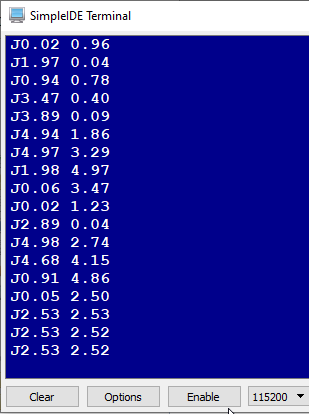If the first character is not a "C" or a "J" then the transaction is discarded.
If the length of a joystick transaction is not >= 10 it is discarded.
The compass transactions are not working correctly in terms of correct direction.
```/*
mobile.c

Demonstrates using full duplex serial communication to exchange information.
https://www.appconwireless.com/showpro.php?id=94
http://learn.parallax.com/tutorials/language/propeller-c/propeller-c-simple-protocols/full-duplex-serial
https://github.com/parallaxinc/Simple-Libraries/blob/master/Learn/Examples/Protocols/XBee UART Command Mode Example.c
*/

#include "simpletools.h"
#include "fdserial.h"
#include "compass3d.h"

void compass(void *par);
fdserial *robot;
fdserial *term;
unsigned int stack[44 + 128];
static volatile char dataIn;

int main()
{
robot = fdserial_open(9, 8, 0, 9600);  // fdserial *fdserial_open(int rxpin, int txpin, int mode, int baudrate)
pause(1000);
term = fdserial_open(31, 30, 0, 115200);
pause(1000);
//  fdserial_rxFlush(robot);
cogstart(compass, NULL, stack, sizeof(stack));
int n = 0;
char c;
fdserial_rxFlush(robot);

while(1)
{
for (int i = 0; i < 13; i++)
{
dataIn[i] = '\0';
}
n = 0;
c = fdserial_rxChar(robot);
if(c != -1 && (c == 'J'))
{
while (n < 12)
{
dataIn[n] = c;
n++;
c = fdserial_rxChar(robot);
if (c == -1 || c == '\r')
break;
}
if (c == -1)
{
fdserial_rxFlush(robot);
break;
}
if (c == '\r')
{
dataIn[n] = '\0';
}
dataIn = '\0';
if(strlen(dataIn) >= 10)
{
dprint(term, "%s\n", dataIn);
pause(1000);
}
//      dprint(term, "%d\n", strlen(dataIn));
}
else
{
//    dprint(term, "0XAF 0XAF 0X00 0X00 0XAF 0X80 0X20 0X02 00 00 CS 0X0D 0X0A");
fdserial_rxFlush(robot);
}
}
}

void compass(void *par)
{
int x, y, z;                                // Declare x, y, & z axis variables

i2c *bus = i2c_newbus(3, 2, 0);             // New I2C bus SCL=P3, SDA=P2
compass_init(bus);                          // Initialize compass on bus.
fdserial_txFlush(robot);

while(1)                                    // Repeat indefinitely
{
compass_read(bus, &x, &y, &z);            // Compass vals -> variables
float fy = (float) y;                     // Ints to floats
float fx = (float) x;
float heading = atan2(fy, fx) * 180.0/PI; // Calculate heading with floats
if(heading < 0.0)                         // Convert -180...+180 to 0...360
}
}

```
```/*
controller.c

Demonstrates using full duplex serial communication to exchange information.
https://www.appconwireless.com/showpro.php?id=94
http://learn.parallax.com/tutorials/language/propeller-c/propeller-c-simple-protocols/full-duplex-serial
https://github.com/parallaxinc/Simple-Libraries/blob/master/Learn/Examples/Protocols/XBee UART Command Mode Example.c
*/

#include "simpletools.h"
#include "fdserial.h"

void joystick(void *par);
fdserial *robot;
fdserial *term;
unsigned int stack[44 + 128];
static volatile char dataIn;

int main()
{
robot = fdserial_open(9, 8, 0, 9600);  // fdserial *fdserial_open(int rxpin, int txpin, int mode, int baudrate)
pause(1000);
term = fdserial_open(31, 30, 0, 115200);
pause(1000);
cogstart(joystick, robot, stack, sizeof(stack));
int n = 0;
char c;
fdserial_rxFlush(robot);

while(1)
{
for (int i = 0; i < 13; i++)
{
dataIn[i] = '\0';
}
n = 0;
c = fdserial_rxChar(robot);
if(c != -1 && (c == 'C'))
{
while (n < 12)
{
dataIn[n] = c;
n++;
c = fdserial_rxChar(robot);
if (c == -1 || c == '\r')
break;
}
if (c == -1)
{
fdserial_rxFlush(robot);
break;
}
dataIn = '\0';
dprint(term, "%s\n", dataIn);
pause(1000);
}
else
{
fdserial_rxFlush(robot);
}
}
}

void joystick(void *par)
{
//  pause(1000);                                      // Wait 1 s for Terminal app
adc_init(21, 20, 19, 18);                         // CS=21, SCL=20, DO=19, DI=18

float lrV, udV;                                   // Voltage variables
fdserial_txFlush(robot);

while(1)                                          // Loop repeats indefinitely
{
udV = adc_volts(2);                             // Check A/D 2
lrV = adc_volts(3);                             // Check A/D 3

//pause(1000);                                     // Wait 1/10 s
dprint(robot, "J%.2f %.2f\r", udV, lrV);       // Display voltage
pause(1000);                                     // Wait 1/10 s
//    dprint(term, "%.2f %.2f\n", udV, lrV);       // Display voltage
//    pause(1000);                                     // Wait 1/10 s
//    dprint(robot, "%.2f\n", lrV);       // Display voltage
//    pause(1000);                                     // Wait 1/10 s
}
}

```